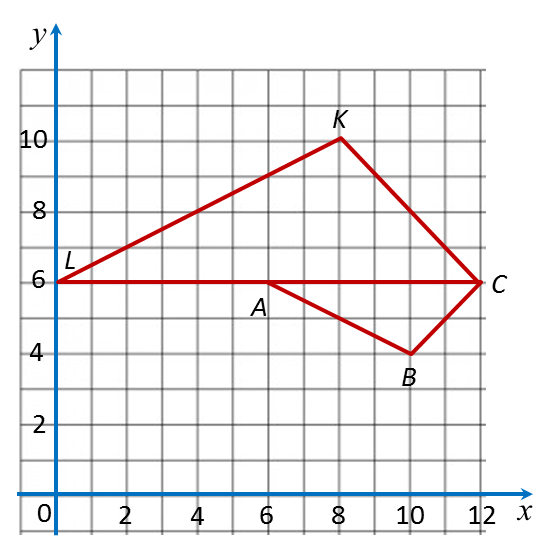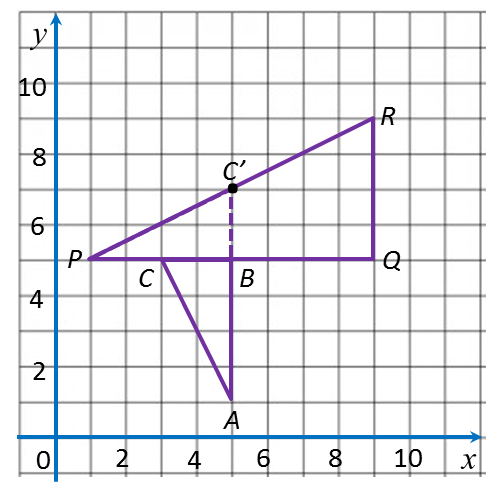# 3.1d Describing the Combination of Two Transformations Given the Object and the Image

3.1d Describing the Combination of Two Transformations Given the Object and the Image

Example 1:In the diagram above, triangle KLM is the image of triangle ABC under the combined transformations VW. Describe in full, the transformation and the transformation V.

Solution:Figure ABC W→ Figure KB’C’ V → Figure KLM

$W=\text{Translation}\left(\begin{array}{l}0\\ 4\end{array}\right)$
= Enlargement with centre (1, 1) and a scale factor of 3.

Example 2:In the diagram above, triangle CKL is the image of triangle ABC under the combined transformations UY. Describe in full, the transformation and the transformation U.

Solution:Figure ABC Y→ Figure AB’C U → Figure CKL
Y = Reflection in the straight line y = 6
= Enlargement with centre (12, 6) and a scale factor of 2.

Example 3:In the diagram above, triangle PQR is the image of triangle ABC under the combined transformations TW. Describe in full, the transformation and the transformation T.

Solution:Figure ABC W→ Figure PBC’ T → Figure PQR
W = Clockwise rotation of 90o about the point (5, 5)
= Enlargement with centre (1, 5) and a scale factor of 2.